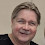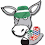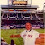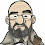## Monday, November 26, 2012

### NFL Picks Contest- Results and Standings

Here are the results and updated standings in the NFL Picks Contest

lightning- Denver (W 17-9) = 18 pts.
TwoMinuteWarning- Denver (W 17-9) = 18 pts.
Grange95-  New England (W 49-19) = 40 pts.
ohcowboy12go- Denver (W 17-9) = 18 pts.
M Prosk- no pick = 0 pts.
Full House- Denver (W 17-9) = 18 pts.
Music City Momma- Cincinnati (W 34-10) = 34 pts.
Coach-  New England (W 49-19) = 40 pts.
Neophyte- Denver (W 17-9) = 18 pts.
Waffles- no pick = 0 pts.
MOJO-  Denver (W 17-9) = 18 pts.
ggrouchie- New England (W 49-19) = 40 pts.
Vegas Vic- New England (W 49 19) = 40 pts.
Captain Crunch- Denver (W 17-9) = 18 pts.

Here are the current standings;

grrouchie - 14+48+20+5+16+40 = 143 pts
Coach - 20+19+22+13+16+40 = 130
MOJO - 40+19+22+13+16+18 = 128
Grange95 - 20+19+22+13+14+40 = 128
Vegas Vic - 16+19+22+13+16+40= 126
TwoMinuteWarning - 13+19+22+16+31+18 = 119
Music City Momma - 17+19+24+5+17+34 = 116
Captain Crunch - 13+11+18+16+31+18 = 107
lightning - 13+19+24+13+16+18 = 103
Neophyte - 17+19+0+13+32+18 = 99
M Prosk - 17+0+41+5+17+0 = 80
Waffles - 15+11+22+16+14+0 = 78
ohcowboy12go - 13+19+22+5+13= 72
Full House - 20+19+22-(34)+16+18 = 61

Congratulations to grrouchie for grabbing the lead this week!

1.Middle of the pack. *sigh*

2.As a Cowboys fan, I feel your pain.

As an Astros fan, I can only dream...

3.What's with all the Steelers fans at the top of the leaderboard?!

1.Well, we aren't there in the NFL so it's all Fantasy Land For US!

Also, I'd like to thank MoJo for NOT picking New England and allowing me to temporarily grab the lead.
I'd like to thank Coach for riding on my coattails to the top of the charts - I'll take that check in small unmarked bills at the poker table :)

4.@ Coach and grrouchie- Did I forget to mention the 20 pt PITA (pain in the ass) penalty for Steelers' fans? That would move you to the middle of the pack :)